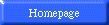Problem by Peter Ash (geometry forum 12 April 2006)

Let P be a point on a circle with center O, and let Q be a point on ray OP, with OQ > OP. Let R and S be the points where the tangents from Q intersect the circle, and let T and U be the midpoints of the segments RQ and SQ. Find the locus traced out by T and U, as Q moves.
The curve looks something like y = x4. Does it have a name? Can anyone find a simple form of the equation for the curve?

Solution

Equation is obtained using the locus equation feature of Cabri-Geometry II Plus.
The figure is built like the Luigi Tomasi's example in geometry forum:
the circle has equation: x2+y2=1, and the point P has coordinates (0; 1).

Click thumbnail image: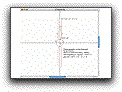Calculating the equation (by François Rideau and Michel Tixier).
Choose parameter t so that R coordinates are R(sin(t);cos(t)). So Q( 0;1/cos(t)) and T( (1/2) sin(t);(1/2)( cos(t) + 1/cos(t)) )
Then elimination of t via sin(t)2 + cos(t)2 = 1 gives cartesian equation 4x4 + 4x2.y2 - 4x2 - y2 + 1 = 0

Alternate cartesian equation (by Michel Tixier):
y = (1-2x2) / √(1 - 4 x2) with x in ] -1/2 , 1/2 [.
x = ±1/2 are two asymptotes of the curve.

Changing radius r = OP for 1,2,3,4,... we can "deduce" with Cabri the general formula:
4x4 + 4x2.y2 - 4r2.x2 - r2. y2 + r4 = 0
Calculating equation as above confirms this result.

Up to our knowledge this curve has not got a special name.
Jean-Marie Laborde proposes a generalization : what happens if you choose another point than the midpoint, e.g. a point at a constant ratio on QR ?
Joseph Hormière found in the case r = 1 the equation x4 + x2.y2– 2k.x2– k2.y2 + k2 = 0 where k is the ratio (k=1/2 gives Peter Ash's case).

François Rideau proposes another problem: the construction of the tangent to the quartic at point T .
This problem is difficult from the mathematical point of view and the confirmation with Cabri is amazing.
Here is the François Rideau's figure: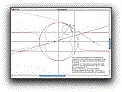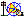Figure (QuartiqueFR.fig) in FrenchFigure (QuarticFR.fig) in English

Other loci can be constructed thanks to F. Rideau's figure. The pedal of the nephroïd is a rose: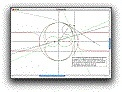Figure (Rosace.fig) in FrenchFigure (Rose.fig) in English

The pedal of the quartic is a non-regular quadrifolium (four-leafed rose):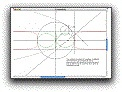Figure (PodaireJB.fig) in FrenchFigure (PedalJB.fig) in English